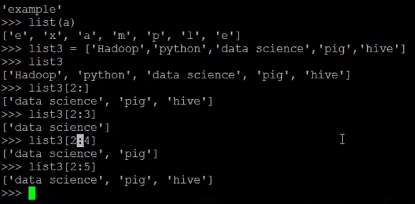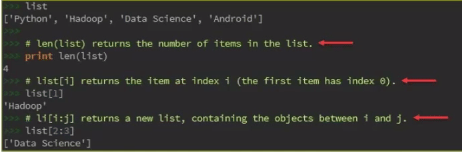# Understanding Range Function and Sequences in Python

Last updated on Jul 15,2021 17.4K Views## Range Function

Range() generates lists containing arithmetic progression.

There are three variations of range() function:

>> range(stop) – Starts from 0 till (stop – 1)

>> range(start,stop) – Ends at (stop – 1)

>> range(start,stop,step) – Step cannot be 0, default is 1

## Example of Range Function

range(stop): If the range is defined as 5, it will simply show the list of numbers falling in the range from 0 to 5. It starts by default from 0 and stops before 5, as defined.

range(start, stop): In this, point of starting as well as stopping is defined. As shown in the example below, the start range has been defined as 5, while stop range as 10. Hence, it will display the numbers 5, 6, 7, 8, 9, which range between 5 to 10.

range(start, stop, step): The first two values defined here are the same, start and stop, while the third one is step, which means the difference between every two consecutive numbers. For example, if range is defined in this way: range(0, 10, 2). It will give away numbers between 0 to 10, but with a difference of 2, in this way: [0, 2, 4, 6, 8]. The step here cannot be given 0 value. It has to be 1 or greater than 1.

## Sequences in Python

A sequence is a succession of values bound together by a container that reflects their type. Almost every stream that you put in Python is a sequence.

Types of Sequences

• Lists
• Tuples
• Xrange
• String

Some of the sequences that Python supports are strings, lists, tuples and Xrange objects. Python has a bevy of methods and formatting operations that it can perform on each of these.

## List

• A list is a sort of container that holds a number of other objects, in a given order.
• The list type implements the sequence protocol, and it also allows you to add and remove objects from the sequence.
• It is an ordered set of elements enclosed in square brackets.

Simple definition of list – li = []

```li = list() # empty list
li = list(sequence)
li = list(expression for variable in sequence)```## Accessing List Elements

To access the elements of a list:

```n = len(li)
item = li[index] #Indexing
slice = li[start:stop] #Slicing```

Example## List Indexing

list[i] returns the value at index i, where i is an integer. A negative index accesses elements from the end of the list counting backwards. The last element of any non-empty list is always li[-1]. Python raises an IndexError exception, if the index is outside the list.

Got a question for us?? Mention them in the comments section and we will get back to you.Related Posts

Command Line Arguments in Python

Exception in Python

Python 101: Hello World Program

Start your Training in Python today!REGISTER FOR FREE WEBINARThank you for registering Join Edureka Meetup community for 100+ Free Webinars each month